2019-06-03 16:08:47

### 1 SPL中的序列与集合

SPL中，序列如同整数、字符串一样是非常常用的基本数据类型，也能进行相应的基本运算。从集合角度出发，SPL提供了两个集合AB的交、并、联、差等基本运算符：A^BA|BA&BA\B等。如果能够从这些运算开始深刻理解并熟练运用，解决问题时就能更主动地采用集合思维，从而充分利用已知的数据，思路更直接和简洁，方法也更加简易清晰。

A
1
=demo.query("select EID, NAME,   SURNAME, GENDER, STATE from EMPLOYEE")
2
=A1.select(GENDER=="M")
3
=A1.select(STATE=="California")
4
=A2^A3
5
=A1.select(GENDER=="M" &&   STATE=="California")
6
=A2&A3
7
=A1.select(GENDER=="M" ||   STATE=="California")
8
=A2\A3
9
=A1.select(GENDER=="M" &&   STATE!="California")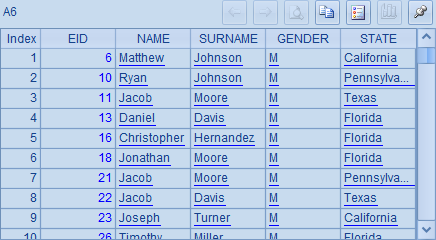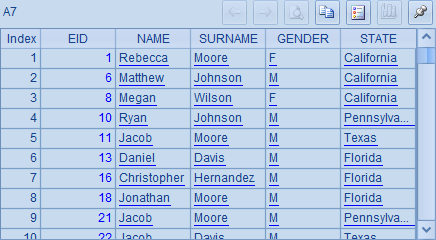A
1
[1,2,3,4]
2
[1,3,3,2]
3
=[1,2,3]==[1,3,2]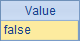A
1
[1,2,3]
2
[3,1,5]
3
=A1^A2
4
=A2^A1
5
=A1&A2
6
=A2&A1

A3,A4,A5A6中的计算结果依次如下：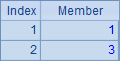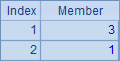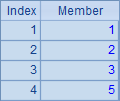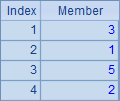A
1
=[1,2,3]==[3,2,1]
2
=[1,2,3]==[3,2,1].sort()
3
=[1,2,3].eq([3,2,1])
4
=[1,2,3].eq([3,2,2])
5
=[1,2,2,3].eq([3,2,1,2])
6
=[1,2,2,3].eq([3,2,3,1])

A1A2中判断两个序列是否相同，结果如下：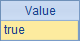A3A4A5A6分别都使用函数A.eq(B)来判断两个序列是否有着同样的成员，结果依次如下：### 2 循环函数

A
1
[3,4,1,3,6]
2
=A1.sum()
3
=A1.avg()
4
=A1.max()-A1.min()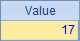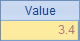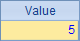A
1
[3,4,1,3,6]
2
=A1.sum(~*~)
3
=demo.query("select * from   EMPLOYEE")
4
=A3.min(~.BIRTHDAY)
5
=A3.min(BIRTHDAY)
6
=A3.avg(interval@y(BIRTHDAY,HIREDATE))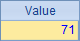A4A5A6则对A3生成的集合中的每个成员的属性值进行循环计算。A3对员工信息序表进行查询后生成集合，其中每个成员是一个员工的信息。A4中计算员工最早的生日，即成员生日的最小值，结果如下：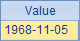A4表达式中的~.可以省略，写成A5的样子，因此计算结果与A4相同。

A6中计算所有员工平均入职年龄，即每个成员入职时间和生日时间的年份差的平均值，结果如下：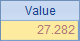1)        先根据参数表达式对集合中的每个成员进行计算，结果称为计算列

2)        再对计算列做聚合计算。

A
1
[A,B,C]
2
[a,b,c]
3
=A1.(A2.(~/~))
4
=A1.(A2.(A1.~/~))
5
=A1.(A1.(A1.~/~))
6
=A1.((arg=~,A1.(arg/~)))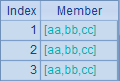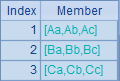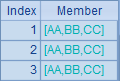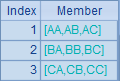### 3 循环次序

A
1
[1,3,2,5,4,8,7]
2
0
3
=A1.(A2=A2+~)
4
[1,1,0,0,1,0,0,0,1,0,1,0,0,0]
5
0
6
=A4.max(if(~==0,A5=A5+1,A5=0))

A3中，通过循环，计算出A1中成员累积和序列：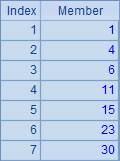A6中，计算出序列A4中，成员0连续出现的最长个数：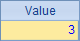### 4 计算序列

A
1
[1,2,3]
2
=A1.(~*~)
3
=A1.(~)
4
=A1.()
5
=A1.(1)
6
=A1.(if(~%2==0,~,0))
7
I love you
8
=len(A7).(mid(A7,~,1))
9
=A8.count(~=="o")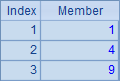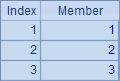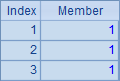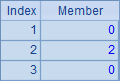A8的完整写法是=to(len(A7)).(mid(A7,~,1))，其中to(n)函数生成一个从1n的数字组成的新序列（熟练后和前面的符号 ~ 一样，有些情况可以省略），对这个序列进行循环，逐个取出A7中的字符串，从而展开为单字符构成的序列；A9计算出其中字母o出现的次数。A8A9中的结果如下：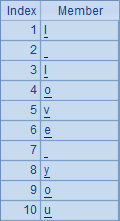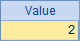A
1
[1,2,3,4,5]
2
=A1.new(~:Origin,~*~:Square)
3
=demo.query("select * from   EMPLOYEE")
4
=A3.new(NAME,age(BIRTHDAY):Age)
5
=A3.new(NAME)
6
=A3.(NAME)

A2根据A1循环计算返回新的序表，其中包含两个字段，一个是A1中的成员，另一个则是该成员的平方值。表达式中的 ~ 前面已经介绍过了，表示当前循环到的序列成员，结果如下：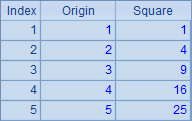A3从数据表EMPLOYEE中取出数据产生一个序表，A4从中获取NAMEBIRTHDAY两个字段，并根据BIRTHDAY计算出该职员的年龄，形成一个新字段Age，最终生成包含了NAMEAge两个字段的新序表。结果如下：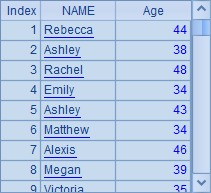A5A6看上去类似，但实际上却有区别，A5A3序表中取出NAME字段，然后直接生成包含了一个NAME字段的新序表；而A6则是根据A3中序表循环计算出由NAME字段构成的序列，两个结果的不同在于，序表有数据结构而序列无数据结构：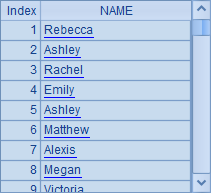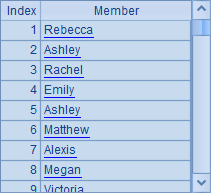A
1
=demo.query("select * from   EMPLOYEE")
2
=A1.new(NAME,age(BIRTHDAY):Age)
3
=A2.run(Age=Age+1)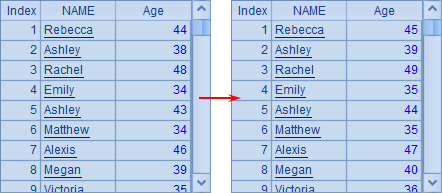### 5 不纯的集合

SPL不要求序列成员的数据类型一致，因此完全可以把数值、字串以及复杂的记录作为同一个序列的成员。

A
1
[1,a3,2,5.4,\$[4.5],2011-8-8]
2
=[A1,4]

A1中包含多种数据类型的成员，而A2中的序列是由序列A1与整数成员构成的，A1A2中的数据如下：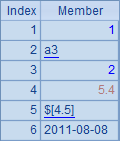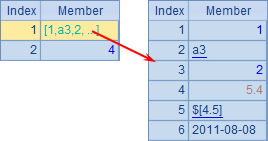A
1
=demo.query("select * from   EMPLOYEE")
2
=demo.query("select * from   FAMILY")
3
=A1|A2
4
=A3.count(left(GENDER,1)=="F")

A4计算员工和家属中，女性一共有多少人。即使员工表和家属表结构不同，但只要其中都包含GENDER字段，就可以正常计算。

### 6 集合的集合

A
1
[[1,2,3,4,5],[1,3,5,7,9],[2,3,5,7]]
2
=A1.conj()
3
=A1.isect()
4
=A1.(~.sum())
5
=A1.(~.(~*~))

A1中是一个序列构成的序列。A2,A3,A4A5分别计算A1中序列成员的和序列、交集序列、各个序列求和的结果以及各个系列每个成员的平方构成的序列。计算后，A2~A5中的结果如下：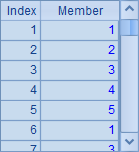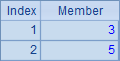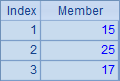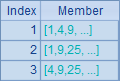A
1
=demo.query("select EID, NAME,   SURNAME, GENDER, STATE from EMPLOYEE")
2
=A1.select(STATE=="California")
3
=A1.select(STATE=="Indiana")
4
=A1.select(STATE=="Florida")
5
=[A2,A3,A4]
6
=A5.(~.count())
7
=A5.(~(1).STATE)
8
=A5.(STATE)
9
=A5.new(STATE,~.count():Count)

A2,A3A4中分别取出California,IndianaFlorida3个州的员工数据。A5中获得的就是由A2~A43个排列构成的序列，是个集合的集合：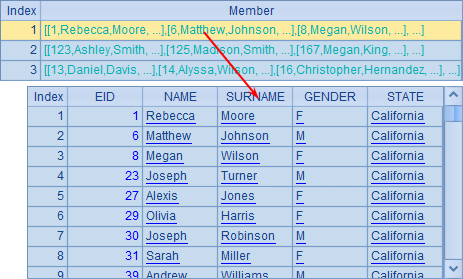A6分别计算各州员工数，结果如下：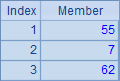A7中取出各个州的名称，表达式中的~(1)是可以省略的，也就是说A8A7是等价的，结果也一样：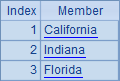A9效果看上去和A6一样，也是统计3个州的员工数，但通过new生成了一个新的序表，看上去更加清晰，也方便以后根据州名检索使用：：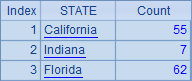### 7 理解分组

SQL正是这样处理的，它的GROUP BY语句总是配合相应的汇总计算。当然，这也是因为SQL自身没有显式的集合数据类型，所以无法直接返回“集合的集合”这类数据，只能把汇总计算强加到分组计算之后。

A B
1
=demo.query("select * from   EMPLOYEE")

2
=A1.group(month(BIRTHDAY),day(BIRTHDAY))
/将员工按生日（月、日）分组
3
=A2.select(~.len()>1)
/有其他人生日与之相同的员工
4
=A3.conj()

5
=A1.group(STATE)
/将员工按所在州分组
6
=A5.new(~(1).STATE:State,~.count():Count)
/用分组结果计算序表，各州员工数
7
=A5.new(STATE,~.avg(age(BIRTHDAY)):Age)
/计算序表，各州员工平均年龄

A B
1
=demo.query("select * from   EMPLOYEE")

2
=A1.group(year(BIRTHDAY))
/按员工出生年份分组
3
=A2.group(int(year(~(1).BIRTHDAY)%100/10))

4
=A2.group(int(year(BIRTHDAY)%100/10))

5
=A2.(~.group(month(BIRTHDAY)))
/把分组后的结果再次分组，A3A4A5都将返回排列的序列

A B
1
\$ select EID,NAME+' '+SURNAME FULLNAME,   DEPT from EMPLOYEE

2
=A1.group(DEPT)
=A2.new(~.DEPT:DEPT,~.count():Count)
3
=A2.sort(~.DEPT:-1)
=A3.new(~.DEPT:DEPT,~.count():Count)
4
=A1.group@u(DEPT)
=A4.new(~.DEPT:DEPT,~.count():Count)
5
=A1.group@o(DEPT)
=A5.new(~.DEPT:DEPT,~.count():Count)

A1中得到的序表如下：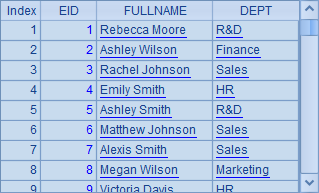A2中按部门名称将员工数据分组，默认情况下，A2中的分组结果就会按照部门名称升序排序。在B列中统计了各种分组情况下各部门的人数，以便通过DEPT列直接查看排序情况。A2B2中结果如下：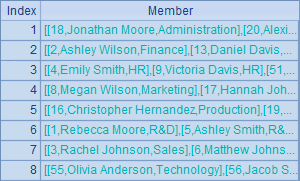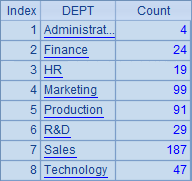A3A2中的分组结果改为按照部门降序排序，效果可以在B3中看到，结果如下：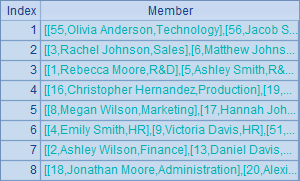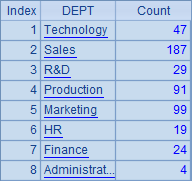A4中添加通过@u选项在分组时保持各部门在员工表中出现的原始顺序。

A5中添加的@o选项指定分组时不对记录做整体排序，而只会将分组表达式相等的相邻记录分为一组，因此更像是“相邻合并”。显然，这种情况可能会出现的“重复”分组。B4B5显示了这两种情况的效果：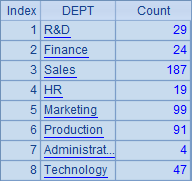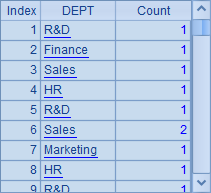### 8 非等值分组

1)        原集合中任何成员都必须在且只能在某一个子集中，也就是分组后的子集成员完全覆盖原集合，并且子集之间没有重叠；

2)        没有空子集

1)        事先指定一组值

2)        将待分组集合中某个表达式计算结果和指定值相同的成员划分到同一个子集

3)        结果的每个子集将和事先指定的值一一对应。

A
1
=demo.query("select * from   EMPLOYEE")
2
[California,Florida,Chicago]
3
=A1.align@a(A2,STATE)

A3中，将集合A1根据A2对齐分组，将A1成员的州名称与A2的成员做对应。在这样的分组过程中，有可能有些员工不在任何一个分组中（其他州的员工），也有可能出现没有任何成员的空组（Chicago不是州名称，根本没有对应的员工）。例如，在某种数据情况下，A3结果：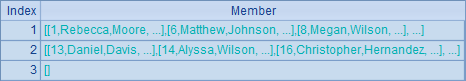A
1
=demo.query("select EID, NAME,   SURNAME, GENDER, BIRTHDAY from EMPLOYEE")
2
[?<=35,? <=45,?>45]
3
=A1.enum(A2,age(BIRTHDAY))
4
[?<=35,?>20 && ?<=45,?>45]
5
=A1.enum@r(A4,age(BIRTHDAY))

A3中，根据A2中的年龄条件序列进行枚举分组。enum()函数不指定选项时，不允许分组结果出现重复，也就是说A1中某个员工的记录可以不在任何分组中（不过例子中的条件是全覆盖的），但是不会同时出现在两个分组中（也就是不会重叠）。A3结果如下：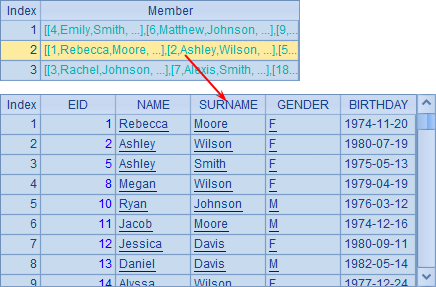A5也是根据A4中的条件序列进行枚举分组，不过这里使用enum函数时添加了@r选项，表示分组时可重复。此时，某个员工的记录有可能同时出现在多个分组中了。例如：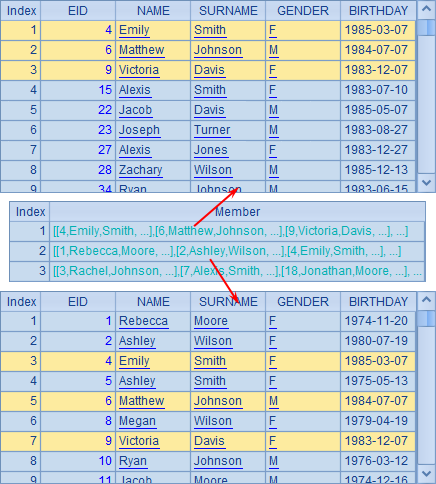align@a函数和enum函数虽然看起来和group函数相差很大，不过在理解了分组运算的本质后，就能明白它们其实都是在做同一件事：即把一个集合拆分成若干个子集，所不同的只是拆分的具体条件和规则不同罢了。